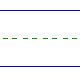Name: ______________________________________________ Date: _____________

Instructions: Find the sum.

 0 + 0 =5 + 0 =2 + 2 =3 + 0 =0 + 1 =4 + 0 =2 + 3 =2 + 1 =0 + 3 =3 + 2 =3 + 1 =1 + 3 =1 + 4 =0 + 4 =2 + 0 =4 + 1 =1 + 1 =0 + 2 =